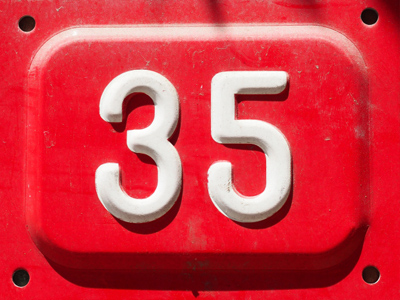5 x 7 = 35.

# 7 Times Table

Learning the times tables is a vital tool when multiplying numbers. By the end of Year 2 children are often fluent in their times tables up to 10. When they enter into KS2 in Year 3 they will also be taught some higher times tables in their Maths and Numeracy lessons. By the end of KS2 they will be familiar with all of the times tables from one to twelve. This quiz will test their knowledge of the 7 times table.

Multiplying numbers by 7 is what learning the 7 times table is about! You may wish to never see a times table again...but keep on practising until you know every answer automatically. Learning your times tables will be beneficial in the long run - you'll find multiplying numbers much easier!

See how well you remember your 7 times table by playing this quiz.

1.
6 x 7 =
28
34
42
43
2.
12 x 7 =
77
84
88
92
10 x 7 = 70 and 2 x 7 = 14. 70 + 14 = 84
3.
2 x 7 =
9
12
14
17
14 days is two weeks, also known as a fortnight
4.
9 x 7 =
60
61
63
65
To times a number by 9 first times it by 10 and then take it away. So, 7 x 10 = 70 and 70 - 7 = 63
5.
8 x 7 =
15
50
53
56
To work out 8 times a number, first times it by 10 and by 2. Then take away the x2 from the x10. So 7 x 10 = 70 and 7 x 2 = 14. 70 - 14 = 56
6.
4 x 7 =
26
28
32
33
7.
7 x 7 =
47
49
51
52
8.
5 x 7 =
12
17
27
35
9.
11 x 7 =
17
70
75
77
10 x 7 = 70 and 70 + 7 = 77
10.
3 x 7 =
18
20
21
23
21 is 7 + 7 + 7 or 3 + 3 + 3 + 3 + 3 + 3 + 3
You can find more about this topic by visiting BBC Bitesize - 7 times table and division facts

Author:  Amanda Swift